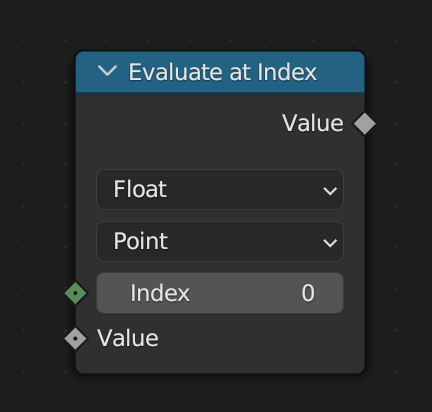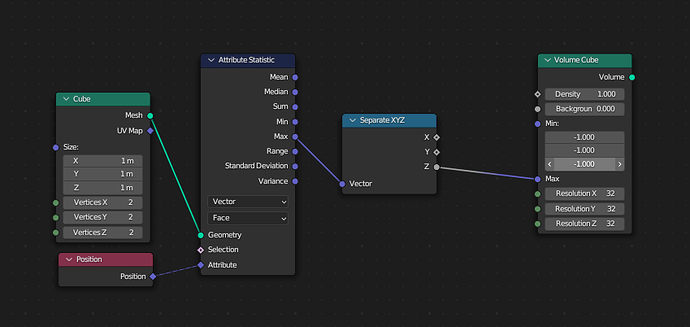# Make Attribute Statistic to Field node

Evaluating statistics on a group of points would be very handy for particle simulations!
F.e. finding the average velocity/positions of a group of nearest points.

In order to maintain backward compatibility, we have to have a way to evaluate the statistics with a geometry input and give a single value rather than a field. That could be done with a node group though, like was suggested above. My point is that we shouldn’t have an option to toggle between the old and new behavior on the same node, because the interface of a node with a geometry input and a field node are very different.

1 Like

“evaluate statistic” will be equal to a evaluate at indexI think a sample index equivalent would be better.Not 100% sure but a evaluate index node would fail when we would convert this setup, because no Geometry would be specified when the Attr. statistic node returns a field.

Right, I think the “Sample Index” node would be more useful for this conversion, since it has a geometry input.

Okay,
thanks for the feedback to all.
I‘ll try to convert the attribute statistic node go fields over the next weeks. After that the legacy conversion and a sample statistic node or something like that.